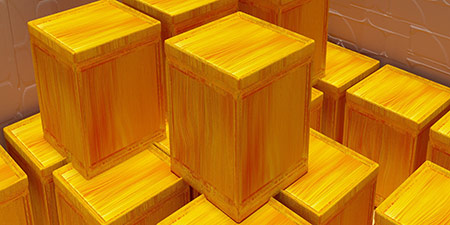# US Gallon (US gal) to Liter (l)

Volume ﹣ Converter US Gallon to Liter

Here you can convert the Volume unit US Gallon into the unit Liter and vice versa you can convert Liter into US Gallon. By clicking the "Swap units" icon, you will always obtain the desired conversion in the calculation result, i.e., US gal to l or l to US gal. With the following calculator you can also calculate any other Volume unit.

The US liquid gallon is defined as 231 cubic inches (inch³) and is equal to 3.785411784 liters.

It is based on a medieval English wine measure. The British gallon, on the other hand, uses a slightly different definition based on beer barrels. There is also a different definition for the American dry gallon.

1 americ. Gallon = 4 americ. Quarts = 8 americ. Pints = 3,785411784 Liter

One liter (l; spelled litre in Commonwealth English and liter in American English) corresponds to one cubic decimeter (dm³) and thus to the volume of a cube with an edge length of 10 cm.

The liter is not part of the International System of Units (SI), but is approved for use with the SI. In addition, the liter is a legal unit of measurement due to national laws.

## Basis for conversion US Gallon (US gal) to Liter (l) and vice versa

The abbreviation for the "Volume unit US Gallon" is US gal. The abbreviation for the "Volume unit Liter" is l.

## Formula for the conversion of US Gallon (US gal) to Liter (l) and vice versa

The calculation from US Gallon to Liter shall be made using the following conversion formula:

Conversion formula US Gallon to Liter

Determine the number of Liter from US Gallon

US Gallon × 3.785411784

## Formula for the conversion of Liter (l) to US Gallon (US gal)

The calculation from Liter to US Gallon shall be made using the following conversion formula:

Conversion formula Liter to US Gallon

Determine the number of US Gallon from Liter

Liter × 0.26417205235815

## Overview table : How many US Gallon are in a Liter ?

US Gallon US gal ⇒ Liter l
0.01 US gal  are  0.03785 l
0.02 US gal  are  0.07570 l
0.03 US gal  are  0.11356 l
0.04 US gal  are  0.15141 l
0.05 US gal  are  0.18927 l
0.06 US gal  are  0.22712 l
0.07 US gal  are  0.26497 l
0.08 US gal  are  0.30283 l
0.09 US gal  are  0.34068 l
0.10 US gal  are  0.37854 l
0.20 US gal  are  0.75708 l
0.30 US gal  are  1.13562 l
0.40 US gal  are  1.51416 l
0.50 US gal  are  1.89270 l
0.60 US gal  are  2.27124 l
0.70 US gal  are  2.64978 l
0.80 US gal  are  3.02832 l
0.90 US gal  are  3.40687 l
1 US gal  corresponds to  3.78541 l
2 US gal  are  7.57082 l
3 US gal  are  11.35623 l
4 US gal  are  15.14164 l
5 US gal  are  18.92705 l
6 US gal  are  22.71247 l
7 US gal  are  26.49788 l
8 US gal  are  30.28329 l
9 US gal  are  34.06870 l
10 US gal  are  37.85411 l
20 US gal  are  75.70823 l
30 US gal  are  113.56235 l
40 US gal  are  151.41647 l
50 US gal  are  189.27058 l
60 US gal  are  227.12470 l
70 US gal  are  264.97882 l
80 US gal  are  302.83294 l
90 US gal  are  340.68706 l
100 US gal  are  378.54117 l
200 US gal  are  757.08235 l
300 US gal  are  1 135.62353 l
400 US gal  are  1 514.16471 l
500 US gal  are  1 892.70589 l
600 US gal  are  2 271.24707 l
700 US gal  are  2 649.78824 l
800 US gal  are  3 028.32942 l
900 US gal  are  3 406.87060 l
1 000 US gal  are  3 785.41178 l

## Overview table : How many Liter are in a US Gallon ?

Liter l ⇒ US Gallon US gal
0.01 l  are  0.00264 US gal
0.02 l  are  0.00528 US gal
0.03 l  are  0.00792 US gal
0.04 l  are  0.01056 US gal
0.05 l  are  0.01320 US gal
0.06 l  are  0.01585 US gal
0.07 l  are  0.01849 US gal
0.08 l  are  0.02113 US gal
0.09 l  are  0.02377 US gal
0.10 l  are  0.02641 US gal
0.20 l  are  0.05283 US gal
0.30 l  are  0.07925 US gal
0.40 l  are  0.10566 US gal
0.50 l  are  0.13208 US gal
0.60 l  are  0.15850 US gal
0.70 l  are  0.18492 US gal
0.80 l  are  0.21133 US gal
0.90 l  are  0.23775 US gal
1 l  corresponds to  0.26417 US gal
2 l  are  0.52834 US gal
3 l  are  0.79251 US gal
4 l  are  1.05668 US gal
5 l  are  1.32086 US gal
6 l  are  1.58503 US gal
7 l  are  1.84920 US gal
8 l  are  2.11337 US gal
9 l  are  2.37754 US gal
10 l  are  2.64172 US gal
20 l  are  5.28344 US gal
30 l  are  7.92516 US gal
40 l  are  10.56688 US gal
50 l  are  13.20860 US gal
60 l  are  15.85032 US gal
70 l  are  18.49204 US gal
80 l  are  21.13376 US gal
90 l  are  23.77548 US gal
100 l  are  26.41720 US gal
200 l  are  52.83441 US gal
300 l  are  79.25161 US gal
400 l  are  105.66882 US gal
500 l  are  132.08602 US gal
600 l  are  158.50323 US gal
700 l  are  184.92043 US gal
800 l  are  211.33764 US gal
900 l  are  237.75484 US gal
1 000 l  are  264.17205 US gal

## Source information

As source for the information in the 'Volume' category, we have used in particular:

## Last update

This page of the 'Volume' category was last edited or reviewed by Stefan Banse on February 20, 2023. It corresponds to the current status.

### Changes in this category "Volume"

• 28.11.2022: Publication of the topic Convert Volumes together with the corresponding texts.
• 28.11.2022: Extension of the Volume Converter by further units: Liquid ounce, cup, tablespoon and teaspoon